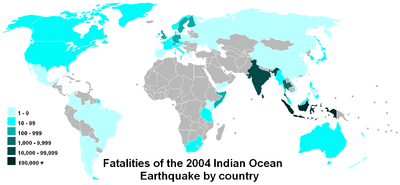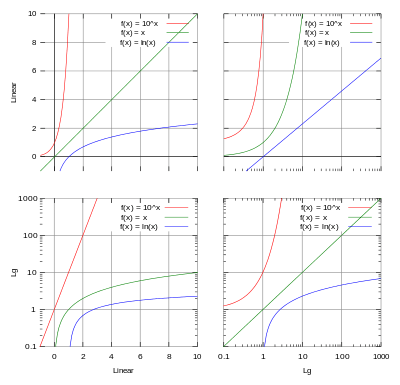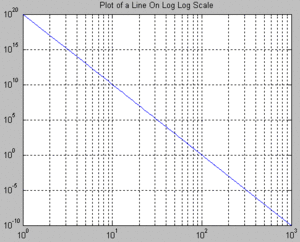# Logarithmic scaleA log scale makes it easy to compare values that cover a large range, such as in this map

A logarithmic scale is a nonlinear scale used when there is a large range of quantities. Common uses include the earthquake strength, sound loudness, light intensity, and pH of solutions.

It is based on orders of magnitude, rather than a standard linear scale, so each mark on the scale is the previous mark multiplied by a value.

## Common usages

The following are examples of commonly used logarithmic scales, where a larger quantity results in a higher value:

The following are examples of commonly used logarithmic scales, where a larger quantity results in a lower (or negative) value:

Some of our senses operate in a logarithmic fashion (Weber–Fechner law), which makes logarithmic scales for these input quantities especially appropriate. In particular our sense of hearing perceives equal ratios of frequencies as equal differences in pitch. In addition, studies of young children in an isolated tribe have shown logarithmic scales to be the most natural display of numbers by humans.It can also be used for geographical purposes like for measuring the speed of earthquakes.

## Graphic representationVarious scales: lin–lin, lin–log, log–lin, and log–log. Plotted graphs are: y = 10 x (red), y = x (green), y = loge(x) (blue).
Main articles: log–log plot and semi-log plot

The top left graph is linear in the X and Y axis, and the Y-axis ranges from 0 to 10. A base-10 log scale is used for the Y axis of the bottom left graph, therefore the Y axis ranges from 0[note 1] to 1,000.

The top right graph uses a log-10 scale for just the X axis, and the bottom right graph uses a log-10 scale for both the X axis and the Y axis.

Presentation of data on a logarithmic scale can be helpful when the data:

• covers a large range of values, since the use of the logarithms of the values rather than the actual values reduces a wide range to a more manageable size;
• may contain exponential laws or power laws, since these will show up as straight lines.

A slide rule has logarithmic scales, and nomograms often employ logarithmic scales. The geometric mean of two numbers is midway between the numbers. Before the advent of computer graphics, logarithmic graph paper was a commonly used scientific tool.

### Log–log plotsPlot on log–log scale of equation F(x) = (x10 )(1020), which can be expressed as the line: log(F(x)) = 10 log(x) + 20.

If both the vertical and horizontal axes of a plot are scaled logarithmically, the plot is referred to as a log–log plot.

### Semi logarithmic plots

If only the ordinate or abscissa is scaled logarithmically, the plot is referred to as a semi logarithmic plot.Wikimedia Commons has media related to Logarithmic scale.